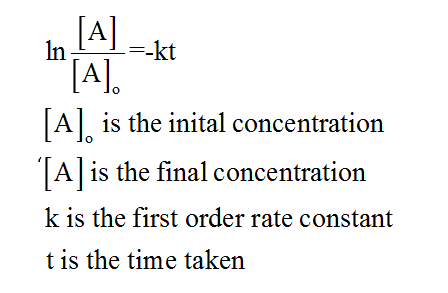# How many seconds will it take for the [C5H6] to be 0.110 M? The k for the reaction is 0.207 s–1. Cyclopentadiene (C5H6) reacts with itself to form dicyclopentadiene (C10H12). A 0.880 M solution of C5H6 is monitored as a function of time as the reaction proceeds. A graph of ln[C6H5] versus time yields a straight line.

Question
210 views

How many seconds will it take for the [C5H6] to be 0.110 M? The k for the reaction is 0.207 s–1

Cyclopentadiene (C5H6) reacts with itself to form dicyclopentadiene (C10H12). A 0.880 M solution of C5H6 is monitored as a function of time as the reaction proceeds. A graph of ln[C6H5] versus time yields a straight line.

check_circle

Step 1

The rate of a given reaction can be defined as the speed at which the conversion of reactants into the products takes place.

Step 2

The term first order reaction is defined for those reactions which proceed at a rate that has a linear dependence on the concentration of only one reactant.

Step 3

In the given problem, it has been given that the graph of ln C5H6 versus t is a straight line and the rate constant is given to be 0.207 s-1 which means that the given reaction follows the first order kinetics, therefore the equation is as follows:...

### Want to see the full answer?

See Solution

#### Want to see this answer and more?

Solutions are written by subject experts who are available 24/7. Questions are typically answered within 1 hour.*

See Solution
*Response times may vary by subject and question.
Tagged in